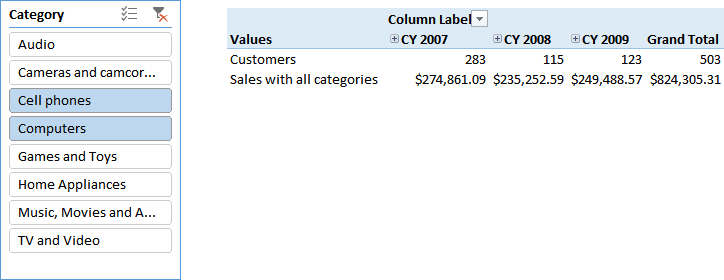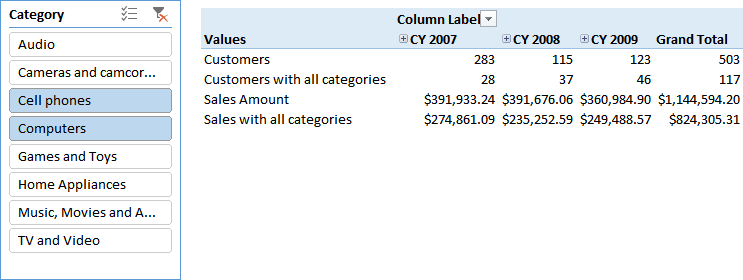Slicers and filters in a report provide a user interface to select one or more items from an attribute, which is applied as a filter to the measures used in the report itself. In case the user selects two or more items from the same slicer or filter, each measure evaluates a selection applying a logical OR condition between the selected items.

For example, consider the following two measures.

```Customers := DISTINCTCOUNT( Sales[CustomerKey] )

Sales Amount := SUMX ( Sales, Sales[Quantity] * Sales[Unit Price] )
```

If you select two product categories in a slicer like in the following example, the result is the number of customers that bought any product of the selected categories (Cell phones, Computers, or both). The measure Sales Amount shows the value of these sales.If you want to create a measure that consider only those customers that bought at least one product for each selected category, you have to apply a filter over Customers based on the selection made on the Category attribute. The technique is based on a filter that evaluates the number of product categories purchased by each customer, and considers only those who have the same number of categories selected by the user.

```Customers with all categories :=
COUNTROWS (
FILTER (
VALUES ( Customer[CustomerKey] ),
"Categories", CALCULATE (
COUNTROWS ( VALUES ( 'Product Category'[Category] ) ),
CALCULATETABLE ( Sales )
)
),
[Categories] = COUNTROWS ( VALUES ( 'Product Category'[Category] ) )
)
)
```

The ADDCOLUMNS function creates a temporary table with one row for each customer. The first column, CustomerKey, keeps the lineage necessary to filter the customers in a CALCULATE function. In fact, you can apply the same filter to a CALCULATE statement that evaluates Sales Amount, or any other measure, with the same logic.

```Sales with all categories :=
CALCULATE (
[Sales Amount],
FILTER (
VALUES ( Customer[CustomerKey] ),
"Categories", CALCULATE (
COUNTROWS ( VALUES ( 'Product Category'[Category] ) ),
CALCULATETABLE ( Sales )
)
),
[Categories] = COUNTROWS ( VALUES ( 'Product Category'[Category] ) )
)
)
```

We did not apply this technique to the count of customers because the result of the FILTER function already provides the desired number of customers, so a CALCULATE function for evaluating another measure is not required in that case.

The final result is visible in the following screenshot.This technique has to be applied to individual measures, and requires the definition of an entity that will be used as a filter for other calculations. It is not possible to create a generic calculation that applies the OR condition in multiple selections on any slicer and for any existing measure.

ORReturns TRUE if any of the arguments are TRUE, and returns FALSE if all arguments are FALSE.

`OR ( <Logical1>, <Logical2> )`

ADDCOLUMNSReturns a table with new columns specified by the DAX expressions.

`ADDCOLUMNS ( <Table>, <Name>, <Expression> [, <Name>, <Expression> [, … ] ] )`

CALCULATEContext transition

Evaluates an expression in a context modified by filters.

`CALCULATE ( <Expression> [, <Filter> [, <Filter> [, … ] ] ] )`

FILTERReturns a table that has been filtered.

`FILTER ( <Table>, <FilterExpression> )`